# How to Solve Linear Models in Matlab using GUROBI

There are situations where you might want to use a specific Mathematical Programming solver and you might have to transform the mathematical model to comply with the API specifications of that Solver. Here I want to show you, how to adapt a Linear Model in order to run it within Matlab using the Gurobi solver.

### A simple Integer Linear Program

To show, what transformations have to be applied in order to migrate a linear model from matlab to Gurobi using the matlab-gurobi API, let’s start with a simple linear model. Let’s say, we have to solve the following integer linear model: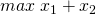s.t.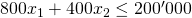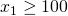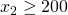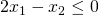In matrix notation we’d have: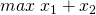s.t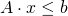Since we have only 2 decision-variables here, we can easily solve the model graphically:

We can see, that the objective function reaches it’s maximum for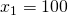and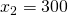.

### Solve the Model with Matlab

In order to solve this integer linear program in Matlab we would have to apply the following modifications:

• Multiply the objective function by (-1), since we want to do maximization, whereas matlab always does minimization (and therefore also multiply the objective value of the result by (-1))
• Convert all constraints showing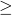signs to constraints showing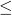signs.
• Provide the constraints being equations in the specific arguments provided (you don’t have to convert equations to combinations ofand)
% objective function vector without constant-term
f = [-1, -1]';
% indexes of integer decision variables
intcon = [1, 2]';
% A-Matrix
A = [800, 400;-1, 0; 0, -1; 2, -1];
% RHS vector
b = [200000, -100, -200, 0]';
% solver call (
x = intlinprog(f,intcon,A,b)
[colX,numFval,numExitflag,sOutput] = intlinprog(f,intcon,A,b)

There are different versions of the intlinprog – command providing additional arguments, details see here. The matlab output after running the script above would look something like this:

Optimal solution found.

Intlinprog stopped at the root node because the objective value is within a gap tolerance of the optimal value,
options.AbsoluteGapTolerance = 0 (the default value). The intcon variables are integer within tolerance,
options.IntegerTolerance = 1e-05 (the default value).

colX =
100
300
numFval =
-400

As we can see, we have to multiply numFval by (-1) to get the correct output-value of the objective function, since we negated the objective-function to be able to transfer the problem into a minimization problem.

### Solve the Model with GUROBI

In order to have the GUROBI commands available in Matlab, you first need to have a working GUROBI Solver installation, a valid license and have run the matlab_setup.m Matlab – Script, coming with GUROBI Solver. See this post, to find a detailled overview on the steps necessary to install GUROBI Solver as well as how to obtain a commercial or academic license.

In order to solve the same model with gurobi, we have to prepare a struct and pass the arguments as described in the reference manual. When consluting the documentation of the matlab API, you realize, that there is no possibility to provideorconstraints in the model, only ‘<‘, ‘>’ and ‘=’ are allowed. (in the documentation it is even stated to accept only ‘=’ – constraints).

However, it turns out that ‘<‘ and ‘>’ are takten as ‘‘ and ‘‘, when solving ILPs. First I introduced slack variables to be able to model ‘‘ and ‘‘ constraints but then I realized, that this is not necessary. One can just provide the model as follows:

% objective function
model.obj = [1,1]';
% signs-vector
model.sense = ['<', '>', '>', '<']';
% right hand side
model.rhs = [200000, 100, 200, 0]';
% lower bounds for decision vars
model.lb = [0,0]';
% upper bounds for decision vars
model.ub = [1e22, 1e22]';
% var types: Integer
model.vtype = ['I', 'I']'
% our matrix
model.A = sparse([800, 400; 1, 0; 0, 1; 2, -1]);
% optimization directive
model.modelsense = 'max';

params.outputflag = 0;
result = gurobi(model, params);
result.x

When running this script, gurobi would deliver the following expected solution:

ans =
100
300

ans =
400